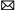# Post Comments

Plz Calculate (3 * 6) =
Our company is planning to program a variable frequency drive for controlling the speed of three phase induction motor. If we vary the amplitude (voltage), what happens to the frequency? Which frequency is suitable for which amplitude?

An induction motor operates at the frequency of the supply feeding it. One may vary the speed by varying the applied frequency.

To operate, the motor requires flux in the iron -- or more specifically in the gap between the rotor and stator. The rotor typically turns at a speed slightly lower than the rotational speed of the stator field (which depends on the frequency). This difference in speed is known as the slip, and is load-dependent (note: AC motors with permanent rotor magnets exhibit no-slip (synchronous) operation). The slip causes the flux to cut through the rotor windings, inducing a current to flow in the rotor. The frequency of the current flow is equal to the slip frequency and can never be zero.

The induction motor is designed as a compromise between iron loss and copper loss. If you reduce the turns on the stator, the copper loss would decrease due to lower resistance, but the flux in the iron would increase causing an increase in the iron loss. If the flux in the iron is too high, the losses increase dramatically and the iron is said to be saturated. The goal is to operate close to saturation to minimize the copper loss but not in saturation to minimize the iron loss. The rated voltage reflects the optimum flux at the rated frequency. As you reduce the frequency applied to the motor, you need to reduce the voltage in order to retain the same flux density. If you don't , the flux density will increase and the iron will saturate.

The variable frequency drive would normally exhibit a constant voltage/frequency ratio from the rated frequency down to a low frequency. In simple terms, you can draw a straight line for zero volts at zero Hz up to rated volts at rated frequency.

At low frequencies, with a constant voltage/frequency ratio, the flux will decrease due to the ratio between the reactive component and the resistive component of the stator; therefore, it's normal to provide some voltage (amplitude) boost at very low frequencies to increase low-frequency torque. This is only necessary for stubborn loads.

Essentially, if you look at the equivalent circuit of an induction motor, you want to keep the current flowing through the magnetizing coil constant at all frequencies. This will provide constant flux.Servo Motor & Drivesbuy@servomotor.cotech@servomotor.co

Buy Servo Motors on ATO.com
Category
Featured